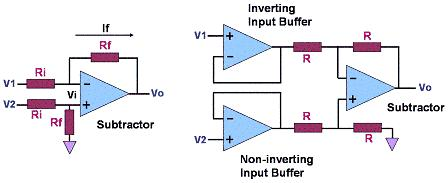Instrumentation Amplifiers (In Amps)

An Instrumentation Amplifier, or In-Amp, is a closed-loop, differential-input amplifier with an output that is single-ended with respect to a reference terminal.  It has closely-matched input resistances that are very high in value, typically greater than 109 ohms. Like an operational amplifier, an instrumentation amplifier must have very low input bias currents (currents flowing in or out the input terminals, typically in the order of several nA for in amps), and a very low output impedance (nominally a few milliohms at low frequencies).

The main difference between an instrumentation amplifier and an operational amplifier is the fact that an op amp is an open-loop device, whereas an in-amp comes with a preset internal feedback resistor network that is isolated from its input terminals.

Because it is an open-loop device, an op amp's function and gain is set by providing it with external components that generally constitute a feedback circuit between its output and its inverting input. On the other hand, the gain of an in-amp, which is used primarily as an amplifier of low-level signals in noisy environments, is either manufacturer-preset or may be set by the user using an external gain resistor or by manipulating internal resistors via some of the in-amp's pins.

Of course, an operation amplifier may be utilized as an instrumentation amplifier. This is done by configuring it as a differential amplifier or subtractor, as shown in Figure 1a, the circuit of which gives an output voltage Vo that is proportional to the difference between the input voltages, or Vo = Rf/Ri (V2-V1).  This equation may be derived as follows:

1)  Vo = -If Rf + Vi where Vi is the voltage at either input of the op amp;

2) but If = (V1-Vo)/(Ri+Rf) and Vi = V2(Rf/(Rf+Ri));

3) thus, Vo = (Vo-V1)(Rf/(Rf+Ri)) + V2 (Rf/(Rf+Ri));

4) or VoRf + VoRi = (V2-V1)Rf + VoRf;

5) simplifying, Vo = Rf/Ri (V2-V1).

A single op amp configured as a subtractor is capable of serving as an in amp for modest applications, i.e., it can amplify the difference between very small signals and reject those that are common-mode (or common to both inputs). This circuit, however, suffers from certain limitations. Commercially available instrumentation amplifier IC's employ multiple internal op amps to overcome these limitations and provide a much superior differential amplification performance.

Figure 1b shows an example of a simple in-amp circuit consisting of multiple op amps (three op amps, to be exact), two of which are used for input buffering with the third one acting as the subtractor itself. Input buffering eliminates problems associated with relatively low input resistances or resistance mismatch between inputs.Figure 1. a) an operational amplifier configured as a differential amplifier to serve as a simple in-amp (left); b) an in-amp consisting of three (3) op amps (right)

Properties that define a high quality instrumentation amplifier are: 1) high common mode rejection ratio; 2) low offset voltage and offset voltage drift; 3) low input bias and input offset currents; 4) well-matched and high-value input impedances; 5) low noise; 6) low non-linearity; 7) simple gain selection; and 8) adequate bandwidth.

Examples of applications where in-amps may be used include: 1) data acquisition from low output transducers; 2) medical instrumentation; 3) current/voltage monitoring; 4) audio applications involving weak audio signals or noisy environments; 5) high-speed signal conditioning for video data acquisition and imaging; and 6) high frequency signal amplification in cable RF systems.